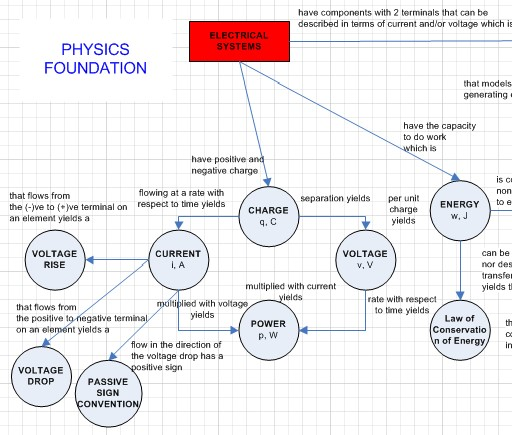# Electrical Engineering: AC & DC Circuits

Resources of all types for electrical engineering.

## Direct Current Circuits

DC Circuits

Circuit Quantities

This content will be helpful for Physics II (PH112), Electrical Systems (ES203), and DC circuits (ECE203).Circuit Elements and Ohm’s Law

This content will be helpful in Physics II (PH112), Electrical Systems (ES203), and DC circuits (ECE203).Kirchhoff’s Laws

This content will be helpful in Physics II (PH112), Electrical Systems (ES203), and DC circuits (ECE203).

Equivalent Resistance, Inductance and Capacitance

This content will be helpful in Physics II (PH112), Electrical Systems (ES203), and DC circuits (ECE203).

Node-Voltage and Mesh-Current Methods

This content will be helpful in Electrical Systems (ES203), and DC circuits (ECE203).Source Transformations

This content will be helpful in Electrical Systems (ES203), and DC circuits (ECE203).

Thevenin and Norton Equivalence

This content will be helpful in Electrical Systems (ES203), and DC circuits (ECE203).

Superposition

This content will be helpful in Electrical Systems (ES203), and DC circuits (ECE203).

First and Second Order Circuits

This content will be helpful in Circuits & Systems (ECE205).

RC and RL Circuits

RLC Circuit

## Alternating Current Circuits

AC Circuits

This content will be helpful in Electrical Systems (ES203), and AC Circuits (ECE204)

Phasors

The video at the following links shows the relationship between sinusoids, RMS, and phasors.

Sinusoids, RMS Phasors

Impedance

Phasor Analysis

AC Power

This content will be helpful in Electrical Systems (ES203), and AC Circuits (ECE204)

Average and Effective Values

Instantaneous, Average and Reactive Power

Complex Power

Operational Amplifiers

This content will be helpful in Electrical Systems (ES203), DC Circuits (ECE203), AC Circuits (ECE204) and Circuits & Systems (ECE205).

Laplace Analysis

This content will be helpful in Circuits & Systems (ECE205).

Laplace Transforms

Inverse Laplace Transforms

Laplace Circuit Analysis

Initial and Final Value Theorem

Filters

This content will be helpful in AC Circuits (ECE204) and Circuits & Systems (ECE205).

High-Pass

Low -ass

Band-Pass

Band Reject

;